# Reconstruction of 3-D Radiation Pattern from 2-D Orthogonal Slices

This example shows how to reconstruct 3-D radiation pattern using `patternFromSlices` function. A 3-D radiation pattern is a very important tool for antenna analysis, characterization, design, planning, and applications. This example shows reconstruction of 3-D radiation from 2 orthogonal slices. Pattern reconstruction for an omni-directional and directional antenna are considered.

### Omni-Directional Antenna

Define an omni-directional antenna such as a dipole with a specific frequency and required elevation and azimuth angle.

```ant = dipole; freq = 70e6; ele = -90:5:90; azi = -180:1:180;```

### Generate Orthogonal 2-D Slices

The slice is along the vertical direction using patternElevation function. Here we can give other 2-D pattern data as well.

```vertSlice = patternElevation(ant,freq,0,Elevation=ele); theta = 90 - ele; ```

Visualize the two orthogonal slices.

```figure patternElevation(ant,freq,0,Elevation=ele);```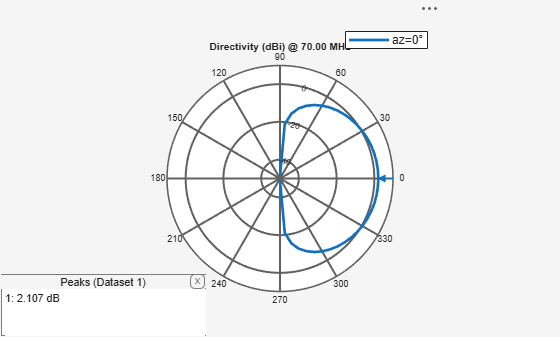```figure patternAzimuth(ant,freq,0,Azimuth=azi);```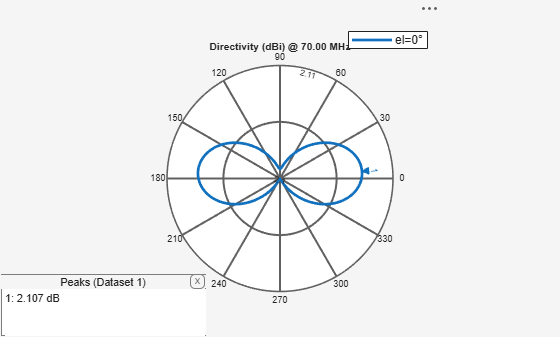### Reconstruction of 3-D Radiation Pattern

For an omni-directional antenna, you can reconstruct the 3-D pattern using `vertSlice` alone. When only the elevation pattern data is provided, function assumes omnidirectionality of the antenna with symmetry about the z-axis (i.e., azimuthal symmetry).

`patternFromSlices(vertSlice,theta);`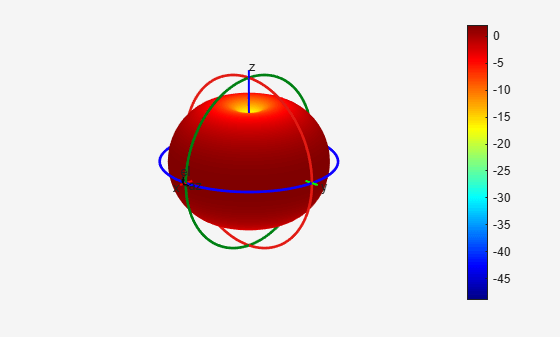Reconstruction using both `vertSlice` & `horizSlice` data points can also be done for the above case. The reconstructed pattern does not vary. Thus for any omni-directional antenna, 3-D pattern can be reconstructed with enough data points from orthogonal slices along the theta direction. The reconstructed radiation pattern looks like the 3-D radiation pattern which can be obtained using the pattern function.

Discarding of data points during reconstruction of 3-D pattern happens when both data points span across 360 degrees in 2-D plane. Since the algorithm need maximum span of 360 degree in one plane and a span of 180 degree in the other plane, extra data points are discarded.

```vertSlice = patternElevation(ant,freq); theta = 90 - (-180:1:180);```

Dimension of `pat3D` is not equal to the `length(phi)*length(theta)` in this case. The size of thetaout also vary from that of theta dimension. Also, `thetaout` data shows the values for which data points have been considered during reconstruction.

`[pat3D,thetaout]=patternFromSlices(vertSlice,theta);`
```Warning: Vertical pattern slice data from backplane for theta greater than 180 degrees is discarded. ```
```dim_theta = size(thetaout); disp(dim_theta);```
``` 1 181 ```

3-D radiation pattern is not affected by data discard since there are enough data points along both the orthogonal planes for reconstruction of 3-D pattern. This result is the same as that of the above reconstructed 3-D radiation pattern.

`patternFromSlices(vertSlice,theta);`
```Warning: Vertical pattern slice data from backplane for theta greater than 180 degrees is discarded. ```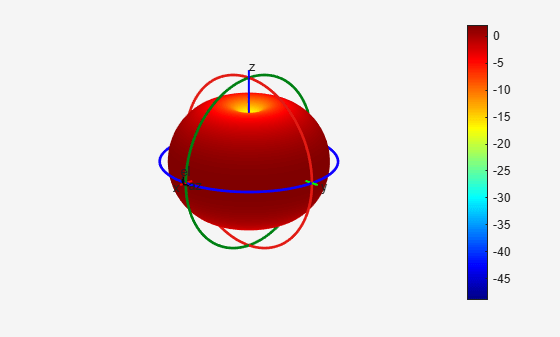### Directional Antenna

Define a directional antenna such as a helix with specific frequency and values for elevation and azimuth angles.

```ant_dir = helix(Tilt=90, TiltAxis=[0 1 0]); freq = 2e9; ele = -90:5:90; azi = -180:5:180;```

### Orthogonal 2-D Slices

The slice along the vertical direction using `patternElevation` function.

```vertSlice = patternElevation(ant_dir,freq,0,Elevation=ele); theta = 90 - ele;```

The slice along the horizontal direction using patternAzimuth function.

```horizSlice = patternAzimuth(ant_dir,freq,0,Azimuth=azi); phi = azi; ```

Visualize the two orthogonal slices.

```figure; patternElevation(ant_dir,freq,0,Elevation=ele);```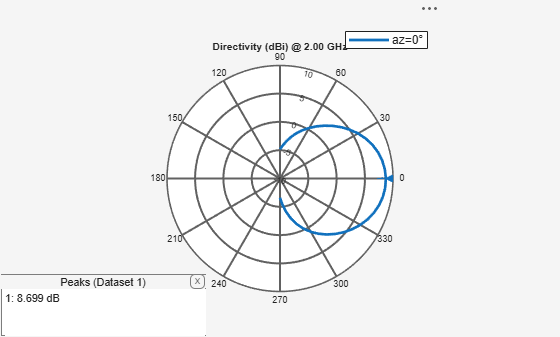```figure; patternAzimuth(ant_dir,freq,0,Azimuth=azi);```### Reconstruction Of 3-D Radiation Pattern

For a directional antenna pattern, both the horizontal and vertical slice must be provided for accurate pattern reconstruction. Two separate algorithms are implemented for pattern reconstruction as below.

#### Summing Method

The "classic" summing algorithm is the default method. This algorithm can be used for near-perfect reconstruction of omni-directional antennas than for directional antenna.

`patternFromSlices(vertSlice,theta,horizSlice,phi);`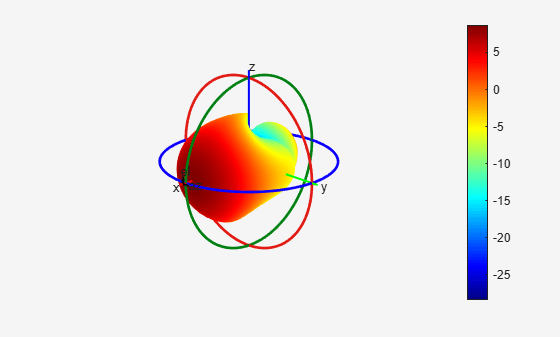#### CrossWeighted Method

In this algorithm, the normalization parameter can be changed to obtain different results for the reconstructed pattern about the estimated directivity/gain.

`patternFromSlices(vertSlice,theta,horizSlice,phi,'Method','CrossWeighted');`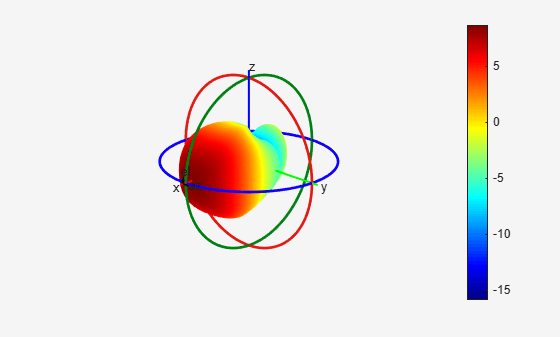### 3-D Radiation Pattern Using `pattern` Function

Visualize the original 3-D radiation pattern of a helix antena using `pattern` function.

```figure pattern(ant_dir,freq);```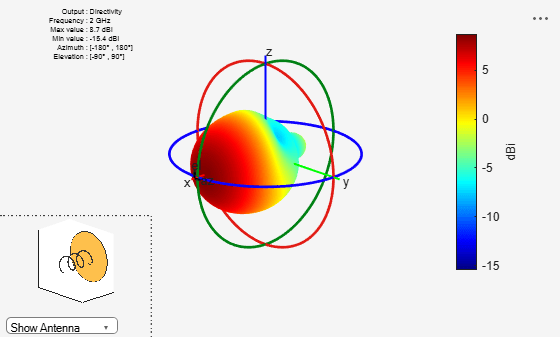By comparing the above 3-D radiation pattern using pattern function with the reconstructed 3-D pattern, it is clear that the front plane of the 3-D pattern is reconstructed well compared to its back plane. Also, when reconstruction is done using CrossWeighted method is more accurate then summing method for this case.

### Read and Visualize Antenna Data from Manufacturer

Antenna manufacturers typically provide details of the antennas that they supply together with the two orthogonal slices of the radiation pattern. The pattern data is available in a variety of formats. One such format that is supported in the Antenna Toolbox is the MSI file format (extension .msi or .pln). Use the msiread function to read the data into the workspace.

`[Horizontal,Vertical,Optional] = msiread("Test_file_demo.pln");`

### Adjust to dBi if data is in dBd

```if strcmpi(Optional.gain.unit,"dBd") Horizontal.Magnitude = Horizontal.Magnitude + 2; Vertical.Magnitude = Vertical.Magnitude + 2; end```

Visualize the vertical and horizontal gain data in an interactive 2-D polar plot.

```figure P = polarpattern(Vertical.Elevation, Vertical.Magnitude); P.TitleTop = "MSI Planet file data"; createLabels(P,"az=0#deg");```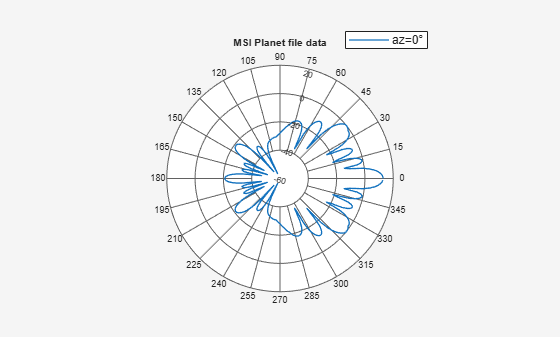```figure Pel = polarpattern(Horizontal.Azimuth, Horizontal.Magnitude); Pel.TitleTop = "MSI Planet file data"; createLabels(Pel,"el=0#deg");```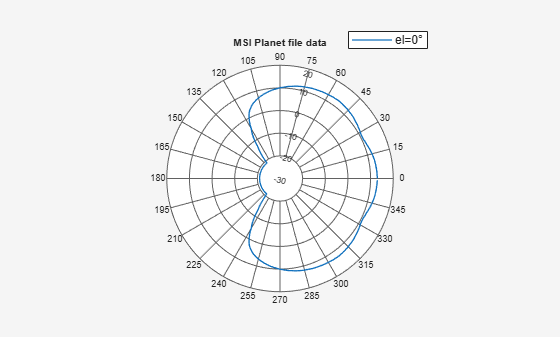### Reconstruction of 3-D Radiation Pattern

Extract the pattern slice magnitude data from the two output structures as well as the azimuth and elevation angle data. Note, that the angle data must be adjusted for the phi-theta convention. The azimuth angles maps to phi but the elevation angle is adjusted by 90 degrees to map to theta.

```vertSlice = Vertical.Magnitude; theta = 90-Vertical.Elevation; horizSlice = Horizontal.Magnitude; phi = Horizontal.Azimuth; patternFromSlices(vertSlice,theta,horizSlice,phi,Method="CrossWeighted");```
```Warning: Vertical pattern slice data from backplane for theta greater than 180 degrees is discarded. ```